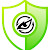## Powerball

FREEMIUM
By Avora Tech | Updated 5 months ago | Entertainment
Popularity

Latency

Service Level

# Powerball API

Powerball API provides the most accurate and up-to-date winning Powerball Lottery numbers. Get more out of your statistics with our unmatched complete and easy -to-use API which separates each winning number so that your statistics can view data for rainbow tables, bell curves, and so much more.

Powerball players may pick five (5) numbers from two separate pools of numbers - five different numbers from 1 to 69 (the white balls) and one number from 1 to 26 (the red Powerball).

Powerball drawings are held every Wednesday and Saturday at 10:59 p.m. ET.

Statistics Overview
The Powerball number matrix was last changed on Oct 7, 2015, and only results since that date are included in these calculations. These statistics do not include numbers drawn in Double Play drawings. Our statistics complies each number position as a separate dataset. All FirstNumber results are a set, as are SecondNumbers, ThirdNumbers, and so forth. Each set’s data includes the minimum (min), maximum (max), mean, median, mode, standard deviation, variance, sample variance, skewness, kurtosis, and lastly each number from 1 to the maximum has a frequency called “occurrences”. Below is a description of each:

min
The min is the lowest number in the dataset. This runs in O(n), linear time, with respect to the length of the dataset.

Example
`min([1, 5, -10, 100, 2]); // -10`

max
This computes the maximum number in an dataset. This runs in O(n), linear time, with respect to the length of the dataset.

Example
`max([1, 2, 3, 4]); // 4`

mean
The mean, also known as average, is the sum of all values over the number of values. This is a measure of central tendency: a method of finding a typical or central value of a set of numbers.

This runs in O(n), linear time, with respect to the length of the dataset.

Example
`mean([0, 10]); // 5`

median
The median is the middle number of a list. This is often a good indicator of ‘the middle’ when there are outliers that skew the mean() value. This is a measure of central tendency: a method of finding a typical or central value of a set of numbers.

The median isn’t necessarily one of the elements in the list: the value can be the average of two elements if the list has an even length and the two central values are different.

Example
`median([10, 2, 5, 100, 2, 1]); // 3.5`

mode
The mode is the number that appears in a list the highest number of times. There can be multiple modes in a list: in the event of a tie, this algorithm will return the most recently seen mode.

This is a measure of central tendency: a method of finding a typical or central value of a set of numbers.

This runs in O(n log(n)) because it needs to sort the dataset internally before running an O(n) search to find the mode.

Example
`mode([0, 0, 1]); // 0`

standard deviation
The standard deviation is the square root of the variance. This is also known as the population standard deviation. It’s useful for measuring the amount of variation or dispersion in a set of values.

Standard deviation is only appropriate for full-population knowledge: for samples of a population, sampleStandardDeviation is more appropriate.

Example
`variance([2, 4, 4, 4, 5, 5, 7, 9]); // 4`
`standardDeviation([2, 4, 4, 4, 5, 5, 7, 9]); // 2`

variance
The variance is the sum of squared deviations from the mean.

This is an implementation of variance, not sample variance: see the sampleVariance method if you want a sample measure.

Example
`variance([1, 2, 3, 4, 5, 6]); // 2.9166666666666665`

sample variance
The sample variance is the sum of squared deviations from the mean. The sample variance is distinguished from the variance by the usage of Bessel’s Correction: instead of dividing the sum of squared deviations by the length of the input, it is divided by the length minus one. This corrects the bias in estimating a value from a set that you don’t know if full.

Example
`sampleVariance([1, 2, 3, 4, 5]); // 2.5`

skewness
Skewness is a measure of the extent to which a probability distribution of a real-valued random variable “leans” to one side of the mean. The skewness value can be positive or negative, or even undefined.

Implementation is based on the adjusted Fisher-Pearson standardized moment coefficient, which is the version found in Excel and several statistical packages including Minitab, SAS and SPSS.

Example
`sampleSkewness([2, 4, 6, 3, 1]); // 0.590128656384365`

kurtosis
Kurtosis is a measure of the heaviness of a distribution’s tails relative to its variance. The kurtosis value can be positive or negative, or even undefined.

Implementation is based on Fisher’s excess kurtosis definition and uses unbiased moment estimators. This is the version found in Excel and available in several statistical packages, including SAS and SciPy.

Example
`sampleKurtosis([1, 2, 2, 3, 5]); // 1.4555765595463122`

Followers: 8
Resources:
Product Website
API Creator:Avora Tech
avoratechnology